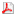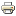Differential equations seminar - December 2, 12pm, lecture room M5Seminar of differential equations will continue on December 2, 2019 at 12pm in lecture room M5.

#### Dr. Rotchana Chieochan (Khon Kaen University, Thajsko)

Floquet theory for q-difference equations

Abstract:

In this talk, we introduce q-periodic functions in quantum calculus and study the first-order linear q-difference vector equation for which its coefficient matrix function is q-periodic and regressive. Based on the new definition of periodic functions, we establish Floquet theory in quantum calculus.

Last Updated on Tuesday, 26 November 2019 12:03